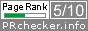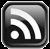LEARN MS-ACCESS TIPS AND TRICKS

Learn Microsoft Access Advanced Programming Techniques, Tips and Tricks.

Time Value Formatting Beyond Twenty Four Hours

Time Format: hh:mm:ss, returns time values up to 23 hours 59 minutes and 59 seconds (23:59:59) and restarts with the value 00:00:01 for next day, 1 second after midnight 00:00:00.  With the built-in Format() Function we cannot format time values beyond 24 hours, like 45 Hours 30 minute and 15 seconds (45:30:15).

Then how do we format time values beyond twenty four hours for applications, like formatting total hours worked by an employee for a week: 40:30:15 or an IT Firm employee's total internet browsing time for the month: 115:47:12, extracted from Server Logs.

We need a function of our own for this purpose.  The following VBA Function: FormatTime() accepts time values in different form, will do the job.  I  have created this function for you and you may Copy Paste the VBA Code into a Standard Module of your project for use.

Public Function FormatTime(ByVal StartDateTime As Variant, Optional ByVal EndDateTime As Variant = CDate(0)) As String
Dim v1 As Double, hh As Integer, nn As Integer, ss As Integer
'-------------------------------------------------------------------------
'Remarks: Time-Format function for time value beyond 23:59:59
'Author : a.p.r.pillai
'Date   : August-2016
'
'Input options
'1. StartDatetime & EndDateTime (both date/time values or both Time Values)
'2. StartDateTime = Date/Time Value and EndDateTime value=0
'3. StartDateTime = Time Value in decimal format – e.g. 0.999988425925926) for TimeValue("23:59:59") or less
'4. StartDateTime = Time Value in Seconds – e.g. 86399 for Timevalue("23:59:59")*86400
'-------------------------------------------------------------------------
'VALIDATION SECTION

'If Input Value is in whole seconds (TimeValue*86400)
'for applications like internet Server log time etc.
'Option4

On Error GoTo FormatTime
If TypeName(StartDateTime) = "Long" Then
GoTo Method2
End If

If EndDateTime > 0 And EndDateTime < StartDateTime Then
'End-Date is less than Start-Date
MsgBox "Error: End-Date < Start Date - Invalid", vbCritical, "Format_Time()"
ElseIf (EndDateTime > StartDateTime) And (StartDateTime > 0) Then
'option 1
If TypeName(StartDateTime) = "Date" And TypeName(EndDateTime) = "Date" Then
v1 = EndDateTime - StartDateTime
GoTo Method1
End If
ElseIf StartDateTime > 0 And EndDateTime = 0 Then
'option 2
'Is it Today's date & Time
If Int(StartDateTime) = Int(Now()) Then
'Remove Date number and take only time value
v1 = StartDateTime - Int(StartDateTime)
Else
'Option 3
'Assumes Value is Time-Value in decimal format
v1 = StartDateTime
End If
End If

'Option 1 to 3
Method1:
hh = Int(v1 * 24)
nn = Int((v1 - hh / 24) * 1440)
ss = Round((v1 - (hh / 24 + nn / 1440)) * 86400, 0)
FormatTime = Format(hh, "00:") & Format(nn, "00:") & Format(ss, "00")
Exit Function

'Time Input in Seconds
'Option 4
Method2:
v1 = StartDateTime
hh = Int(v1 / 3600)
nn = Int((v1 - (hh * 3600#)) / 60)
ss = v1 - (hh * 3600# + nn * 60)
FormatTime = Format(hh, "00:") & Format(nn, "00:") & Format(ss, "00")

FormatTime_Exit:
Exit Function

FormatTime_Err:
MsgBox "Error: " & Err & " - " & Err.Description, , "FormatTime()"
Resume FormatTime_Exit

End Function

Let us try few examples so that you will know how the function parameter values are correctly input to the function.  You may try the following examples by typing them directly on the VBA Debug Window.

The FormatTime() function accepts two Date/Time or Time input parameter values to the function, second parameter is optional.  The following conditions apply when parameter values are passed to the function:

1. When both parameters are entered both should be either Date/Time Values or both Time Values.  Difference of time will be calculated by subtracting first parameter value from second parameter.
2. Second parameter is optional. If omitted then Date value will be ignored when Date/Time value is passed in the first parameter. Time value will be formatted and displayed.
3. First parameter can be time value in decimal format (e.g. 0.999988425925926 ). Omit second parameter.
4. First parameter is acceptable as time value in Seconds (e.g. 86399). Omit second parameter.

Example-1: Both Parameter values are Date/Time Values. Second Parameter value (Date or Time) should be greater than first parameter value.

StartDT = DateAdd("d",-2,Now()) = 8/27/2016 7:38:22 PM

EndDT = Now() = 8/29/2016 7:41:13 PM

? FormatTime(StartDT,EndDT)

Result: 48:02:51

Example-2: First parameter Date/Time Value, second parameter optional and omitted.

SDateTime=Now() or DateVallue("08/29/2016")+TimeValue("18:30:15")

? FormatTime(SDateTime)

Result: 18:30:15

Example-3: First parameter Time Value input as a number (2.00197916667094), the decimal value equal to the first example Result: 48:02:51). When we subtract Start-Date/Time Value from End-Date/Time Value you will get the difference as a decimal number, equal to number of days and time value.  This time number can be the result of summary of time values of several records from a Table or Query. The whole number represents number of days (i.e. 2*24=48 hrs.) + the fractional part in hours:minutes:seconds.

StartDT = 2.00197916667094

? FormatTime(StartDT)

Result: 48:02:51

If the whole number at the left side of the decimal point is equal to the current date number then it will be ignored, when second parameter is omitted.

You can create a time number similar to the one above for testing this function. To do that we will look at few basics on the time value to understand them better.

1 Day = 24 hours. 1 Hour = 60 Minutes. 1 Minute = 60 Seconds. So 1 Day = 24 * 60 * 60 = 86400 Seconds.

1 second before mid-night = 86400-1 = 86399 seconds = 23:59:59

To convert the time 23:59:59 (86399) into internal representation of time value, divide 86400 into 86399 (86399/86400). Result: 0.999988425925926. The time 23:59:59 is stored in computers memory in this form. When combined with the current date number it will be like: 42612.999988425925926 for 08/30/2016 23:59:59

Let us input this time number alone to our function and try out the result.

? FormatTime(0.999988425925926)

Result: 23:59:59

Example-4:

So, if you want to convert a Time number into Seconds then multiply it with 86400. 0.999988425925926 * 86400 = 86399

You can input number of seconds as a time number as first parameter to get it formatted in Hours:Minutes:Seconds.

? FormatTime(86399)

Result: 23:59:59

If you want larger values for testing this function, then try the following examples.

SD = 86399+86400+86000
= 258799 seconds

SD = SD/86400
= 2.9953587962963 time number

? FormatTime(SD)

Result: 71:53:19

Let us input the time value in seconds (let us assume that this value is the summary of Internet browsing time value in seconds).

SD= 258799

? FormatTime(SD)

Result: 71:53:19

If you have a Library Database then you can move this Function into that database so that you don't have to copy the code into all your other databases.

A Library database is a common database you have created with all your custom functions or custom wizards that you can attach with your other Projects. This method will enable you to use these functions in your other projects without duplicating codes in all of them. For a detailed discussion on this subject visit the page MS-Access and Reference Library.

Please leave your comments or suggestions for improvement of FormatTime()  function in the Comments Section, at the end of this Post. Thank you.

Share:

1.In example 2 you show DateValue and TimeValue; where are they defined?

2.DateValue() and TimeValue() are built-in functions. DateValue() function converts date in text format, like "09/15/2016", into a date number 42628. Timevalue converts time in text format, like "12:00:00", into a time number 0.5. When both are added together you can store both this value as a Date/Time number: 42628.5. Date and Time Values are stored in computer's memory in this way. You are using the format() function to display them the way you want it. But, internally the value remains the same without change.

Regards,

Comments are subject to moderation before publishing.

TranslateSubscribe in a readerYour email address:

Delivered by FeedBurner

Featured Post

ROUNDDOWN Function of Excel

We have introduced ROUNDUP() Function of Excel in Microsoft Access earlier.  It works like ROUND() Function except that the number is alway...